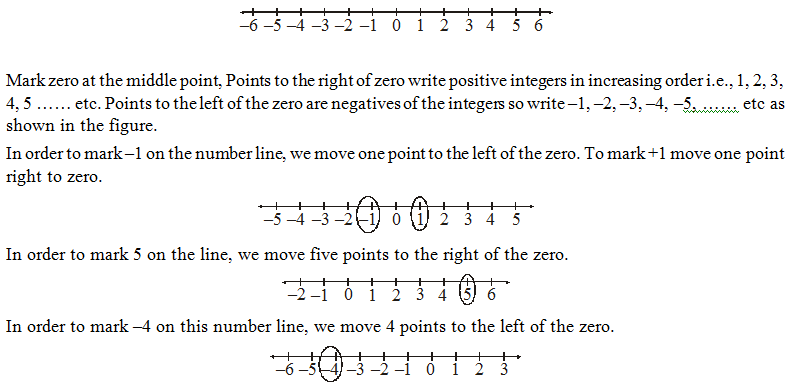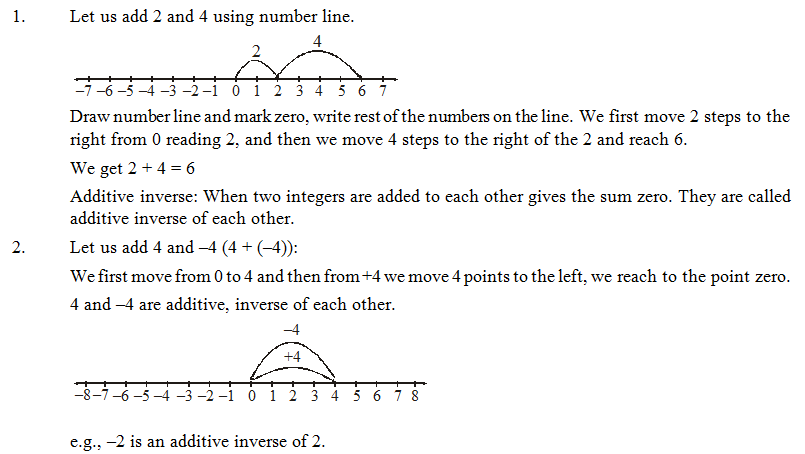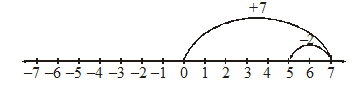# Introduction

## Integers of Class 6

### Integers

Collection of all counting numbers, zero and negatives of counting numbers form the set of integers.

We denote this set with capital alphabet I (or) Z.

I = {0, ± 1, ± 2, ±3, …..}

Note: We use the sign ‘–’ to represent the negatives of the counting numbers.

e.g., –1, –2, –3 etc.

All the negative numbers are less than zero.

Zero is neither negative nor positive.

### Representation of integers on a number line

We can (diagrammatically) represent the integers by drawing a line which never ends and extendable both the sides. Draw a line and make some odd number of points on the line at equal distance.### Addition of integers

We have to add when we have two positive integers or two negative integers, like.

(+5) + (+1) = + 6 or 6

(–5) + (–1) = –6

When the two numbers are negative, answer will take minus (–) sign.

When we have one positive and the negative integer, we must subtract, and answer will take the sign of the bigger integer, ignore the sign of the integers and decide which is bigger and write the sign of that bigger integer to answer.

e.g.,      (–7) + (8) = 1

(–9) + (13) = 4

(8) + (–10) = –2

### Addition of integers on a number lineSubtraction oF integers with the help of number line

We know that to add a positive integer we move towards the right on a number line and for  adding a negative integer we move towards left.

(i)         Let us subtract 2 from 7 (or) 7 – 2 using number line.

Sol.      Rewrite it as 7 + (–2)

We first move 7 points to right of the zero, then we have to move 2 points left from +7. We reach 5.Illustration 1:

Subtract –5 from –10

Sol.      –10 – (–5) = –10 + (additive inverse of –5)

–10 + 5  (since additive inverse of –5 is 5 – (–5) = 5)

Illustration 2:

If 4554 – 3443 = 987 – (–x), then find the value of x.

Sol.      4554 – 3443 = 987 – (–x)

1111 = 987 – (–x)

–x = 987 – 1111                 –x = –124

x = 124

\ the required integer is 124